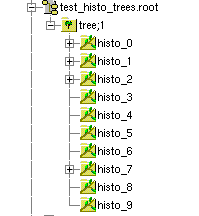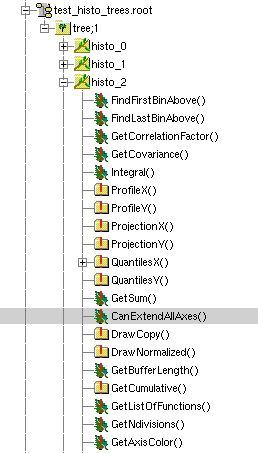# Storing histograms in branches with PyROOT

_ROOT Version: 6.14
_PYTHON Version : 3.6.7
_Platform: Debian 9
_Compiler: GCC 7.3.0

Hello, I’ve written a macro which creates a tree containing 10 branches. Each of these branches is supposed to contain one (and later several) histogram(s). The code below, however, saves the histograms directly into the root file instead of one by one to the branches. Where am I going wrong?

``````from ROOT import TFile, TTree, TH2D, TF2, TFormula
from ROOT import gROOT, gBenchmark

# gBenchmark.Start("hsimple")

# Upper and lower bounds of the 2D histogram and the 2D function:
x0 = 0.
x1 = 100.
y0 = 0.
y1 = 100.

# Simple 2D function to fill the histogram :
xy = TF2( 'xy', '(x+3*y)', x0, x1, y0, y1)

fname = 'test_histo_trees.root'
f = TFile(fname, "RECREATE")
tree = TTree("tree", "Contains the histos. ")

histo = [ TH2D("histo_" + str(i), "example_histo_" + str(i), 100, 0, 100, 100, 0, 100) for i in range(10)]
[histo[i].FillRandom("xy", 1000*i + 100) for i in range(10)]

# histo = TH2D("histo", "example_histo_", 100, 0, 100, 100, 0, 100)

for i in range(len(histo)):
branch_name = 'histo_' + str(i)
tree.Branch(branch_name, histo[i], 'TH2D')
tree.Fill()

tree.Print()

# gBenchmark.Show("hsimple")

f.Write()
f.Print()
f.Close()

input("Press enter to continue. ")
``````

Thanks for the help!

They are likely saved in both places. ROOT saves histograms to the file automatically when you call `f.Write();`.

They are saved directly in the root file and I can see the histograms, as I defined them beforehand, in the TBrowser. When I click on the leafs with the same name as the histograms they do not show the defined histograms but something else.

My question therefore is : Can I save a histogram into a tree in the same way as into a file or does it require a different format?

Hi,

Just to be sure, do you want to have one histogram for each branch and for each entry of the tree? This way you would store num_hists x num_tree_entries histograms in total, num_hists being equal to the number of histogram branches you want to create.

Or instead you want your histograms to be stored as objects in the file, but not belonging to the tree? This way you would store num_hists in total.

Hi etejedor,

for now I would like to store one histogram in each branch. Later, I’d like to add a derived histogram to the same branch (as for instance a radial profile of the original 2D histogram).

But again, let me insist: those histograms that you are going to store, will they be different for each entry of the tree? Or you are just going to store the set of histograms N times?

If you just want to store a set of histograms, do not add them as branches of the tree, just have them in the ROOT file. If at this point you still want to have them as branches, you need to do:

``````# This creates the branches
for i in range(len(histo)):
branch_name = 'histo_' + str(i)
tree.Branch(branch_name, 'TH2D', histo[i]) # note that the second parameter is the class name, not the histo
``````

and then

``````# Note that this will create `num_entries` copies of each of the histograms
for _ in range(num_tree_entries):
tree.Fill()
``````

Okay, I will elaborate. I have about 10 different histograms belonging to a measurement scan, i.e. the histograms are distinct. I want to analyse them once and then store them (and later all the derived histograms like 1D radial projections of the original 2D histograms) into a root file.
With this root file I wish to create graphs without having to repeat the analysis again.
So again - the histogram in histo_0 is different from the one in histo_3 or histo_4 etc.
My idea was to create a tree that contains the histos and the derived histos. Since there are 10 different original histograms, I thought 10 branches were appropriate.

With the modifications you indicated I see the following structure in the TBrowser and I wonder where the histograms are? If I simply save them to a root file I can click on them and see the histogram in the TBrowser window. Below I just see different methods for TH2D. Are the histograms even saved and where?Here is the script with the modifications :

``````from ROOT import TFile, TTree, TH2D, TF2, TFormula
from ROOT import gROOT, gBenchmark, gDirectory

# gBenchmark.Start("hsimple")

# Upper and lower bounds of the 2D histogram and the 2D function:
x0 = 0.
x1 = 100.
y0 = 0.
y1 = 100.

# Simple 2D function to fill the histogram :
xy = TF2( 'xy', '(x+3*y)', x0, x1, y0, y1)

fname = 'test_histo_trees.root'
f = TFile(fname, "RECREATE")
tree = TTree("tree", "Contains the histos. ")

histo = [ TH2D("histo_" + str(i), "example_histo_" + str(i), 100, 0, 100, 100, 0, 100) for i in range(10)]
[histo[i].FillRandom("xy", 1000*i + 100) for i in range(10)]

# This creates the branches
for i in range(len(histo)):
branch_name = 'histo_' + str(i)
tree.Branch(branch_name, 'TH2D', histo[i]) # note that the second parameter is the class name, not the histo

num_tree_entries = 10
for _ in range(num_tree_entries):
tree.Fill()

# # Note that this will create `num_entries` copies of each of the histograms
# for _ in range(num_tree_entries):
#     tree.Fill()

# for i in range(len(histo)):
#     branch_name = 'histo_' + str(i)
#     tree.Branch(branch_name, histo[i], 'TH2D')
#     tree.Fill()

# # tree.Print()

# # gBenchmark.Show("hsimple")

tree.Write()
# f.Write()
# f.Print()
f.Close()

input("Press enter to continue. ")
``````

For this you do not need your histograms to be branches of a `TTree`. You can think of a `TTree` as a table with `k` columns and `n` rows. Even if you have `k` different histograms, you do not need to store them `k * n` times. Therefore, the solution where you just created the histograms and then stored them in the ROOT file, without any tree involved, is the adequate for your use case.

See, for instance, this example:

Can I then think of the root file itself as a dictionary? What you suggested worked. I wrote the histograms, graphs etc. which I stored in a python dictionary directly into the root file :

fname = ‘test.root’
f_write = TFile(fname, “RECREATE”)

``````histo = []
for number in histo_dic :
histo_dic[number]["histo"].Write()
histo_dic[number]["histo_slice"].Write()
histo_dic[number]["tarc"].Write()